# Random projections did it again!

Double Trouble in the Double Descent Curve with Optical Processing Unit.R: “I like it because it is linked with having many classifiers ensembled!” A: “I will pretend I thought about that. Behind many artists maybe there was simply a visionary art critic.” — Just a normal day at work.

The double descent curve [1,2] is the bridge between the classical underparametrized regime and the modern overparametrized one. In earlier blog posts we study the double descent curve and we teach how to recover it.

Despite the staggering success of deep learning, a well-founded theoretical framework to explain it is trudging behind. The remarkable generalization performance of over-parametrized deep neural networks is a puzzle yet to be solved. Nonetheless, there is no shortage of papers about the subject [3, 7, 8, 9, 10].

Modern architectures contain millions of parameters connected in non-trivial ways through a variety of different layers. Such leviathanic objects are hard to examine analytically. How can we partly grasp the essence of such architectures without losing excessive generality and hence allow our friend fond of math to join the party? The answer is random projections.

Random projections for random features regression or random Fourier features have been exploited by many of the aforementioned papers. Despite their simplicity, random features cannot scale up to the demanding request of present-day large data.

LightOn has developed a dedicated hardware, the optical processing unit (OPU), well suited to random projections computations. In some tasks, our co-processor considerably reduces the training time and energy consumption without deteriorating the accuracy of the model when compared with a GPU. A computational time and empirical energy consumption comparison between the OPU and the GPU, performed by , is shown in Figure 1.

A recent paper from Ecole Normale Supérieure  used random projections to provide a theoretical explanation of the double descent curve in the lazy regime — where the weights stay close to their initial value during training. They were able to decompose the different contributions to the test error on synthetic data and gain insight on the double descent curve mystery. In this blog post, we will summarize the novelties discovered by the paper. Next, we will see how we can recover some of the theoretical predictions on real-world data, using a LightOn OPU.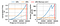Figure 1: Time and energy spent on computing a matrix multiplication (n,D) × (D,D). The batch size n is 3000 (solid line) or 1000 (dotted line). The OPU is compared to an NVIDIA P100 GPU. Plot taken from .

Double, double, toil and trouble.
Fire burn and cauldron bubble.
Double, double, toil and trouble.
Something wicked this way comes!

Harry Potter and the prisoner of Azkaban: Robin Crow / Linsey Williams

The model used by  is the random feature regression: a random matrix multiplication followed by a non-linear function and a ridge regression. The predicted label of a data point x can be expressed as:

Where Θ is a P×D matrix whose elements are sampled from a standard Gaussian, σ is an activation function (ReLu in the paper) and D is the data dimension. The elements of a are determined by the means of ridge regression. The data is generated by sampling from a Gaussian distribution. The labels are given by a linear ground truth corrupted by Gaussian noise:

where τ can be tuned to control the signal to noise ratio (SNR). In Figure 2 a series of data corrupted with a Gaussian with increasing noise.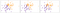Figure 2: Synthetic data generated from a standard Gaussian. The labels are assigned using a ground truth corrupted by Gaussian noise with increasing variance from left to right.

With this model and in the limit of N, D and P going to infinity, while keeping the ratio N/D and P/D constant, the authors were able to analytically isolate the components of the test error: the bias, the initialization variance, the variance induced by the label noise, and finally the variance coming from the data sampling. A visual representation of this decomposition in Figure 3.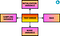Figure 3: Decomposition of the test error.

Their analysis found two smoking guns: a double trouble! Only the noise and initialization variances contribute to the characteristic peak of the double descent curve. The bias and the sampling variances undergo a phase transition at the interpolation point and remain constant after it.

If the initialization variance is contributing in a crucial way to the interpolation peak, then averaging an ensemble of K models with different initialization should mitigate its effect. This intuition has been confirmed in simulation and theory by . Increasing K, the number of averaged models, reduces the influence of the initialization and noise variances by a factor 1/K. Their results are shown in Figure 4 taken from the paper.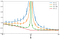Figure 4: The effect of ensembling on the double descent curve. The peak decreases with K until it disappears in the limit of infinite K. Plot taken from .

The random feature regression model can be easily implemented using a LightOn OPU. The data matrix N×D can be randomly projected with an OPU to a higher dimensional matrix N×P. A sketch of the feature regression using an OPU is depicted in Figure 5.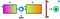Figure 5: the random feature regression implemented with an OPU.

Randomly projecting the data becomes expensive when averaging a high number of models. This is not an issue for the OPU: it is possible to randomly project the data matrix directly to a N×(P×K) matrix in one shot. Then, we can train the various models on slices of this big matrix. The theoretical insights obtained by  can be easily recovered on real data using this algorithm. An animated sketch of this algorithm is shown in Figure 6.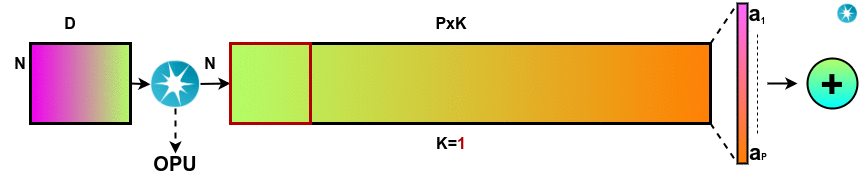Figure 6: We can project our data on the number of random projections * max(K). We can obtain the different values of ensembling by using slices of this matrix.

We were able to recover the mitigating effect of ensembling on MNIST. The plot is shown in Figure 7.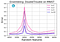Figure 7: The ensembling effect on the double descent curve on a sub-sample of MNIST.

# Conclusion

Random projections proved, once again, to be the fundamental tool to challenge the double descent mystery. In this blog post we have explained the theoretical results of : the double descent peak can be mitigated by averaging an ensemble of models predictions. This is possible because the initialization variance plays a crucial role in the double descent peak. Later we have recovered these results on real-world data. To see the details of how we did it, thanks to our OPU, have a look at the Github repository!

# About us

LightOn is a hardware company that develops new optical processors that considerably speed up big data computation. LightOn’s processors open new horizons in computing and engineering fields that are facing computational limits. Interested in speeding your computations up? Try out our solution on LightOn Cloud ! 🌈

Follow us on Twitter at @LightOnIO , subscribe to our newsletter and register to our workshop series. We live stream, so you can join from anywhere. 🌍

# The author

Alessandro Cappelli, Machine Learning Engineer at LightOn AI Research.

# Acknowledgement

Thanks to Igor Carron, Ruben Ohana, Victoire Louis and Iacopo Poli for reviewing this blog post.

# References

 Geiger, Mario, et al. “Jamming transition as a paradigm to understand the loss landscape of deep neural networks.” Physical Review E 100.1 (2019): 012115.

 Belkin, Mikhail, et al. “Reconciling modern machine-learning practice and the classical bias–variance trade-off.” Proceedings of the National Academy of Sciences 116.32 (2019): 15849–15854.

 Mei Song, and Andrea Montanari. “The generalization error of random features regression: Precise asymptotics and double descent curve.” arXiv preprint arXiv:1908.05355 (2019).

 D’Ascoli, Refinetti, et al. “Double Trouble in Double Descent : Bias and Variance(s) in the Lazy Regime.” arXiv preprint arXiv:2003.01054 (2020)

 Saade, Alaa, et al. “Random projections through multiple optical scattering: Approximating kernels at the speed of light.” 2016 IEEE International Conference on Acoustics, Speech and Signal Processing (ICASSP). IEEE, 2016.

 Ohana et al. “Kernel computations from large-scale random features obtained by optical processing units.” arXiv preprint arXiv:1910.09880 (2020)

 Madhu S Advani and Andrew M Saxe. “High-dimensional dynamics of generalization error in neural networks.” arXiv preprint arXiv:1710.03667, 2017.

 Brady Neal et al. “A modern take on the bias-variance tradeoff in neural networks.” arXiv preprint arXiv:1810.08591, 2018.

 Trevor Hastie et al. “Surprises in high-dimensional ridgeless least squares interpolation”. arXiv preprint arXiv:1903.08560, 2019.

 Preetum Nakkiran et al. “Deep double descent: Where bigger models and more data hurt”. arXiv preprint arXiv:1912.02292, 2019.

We are a technology company developing Optical Computing for Machine Learning. Our tech harvests Computation from Nature, We are at lighton.ai

## More from LightOn

We are a technology company developing Optical Computing for Machine Learning. Our tech harvests Computation from Nature, We are at lighton.ai